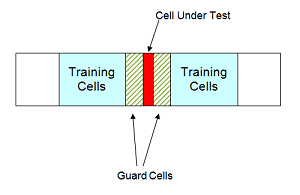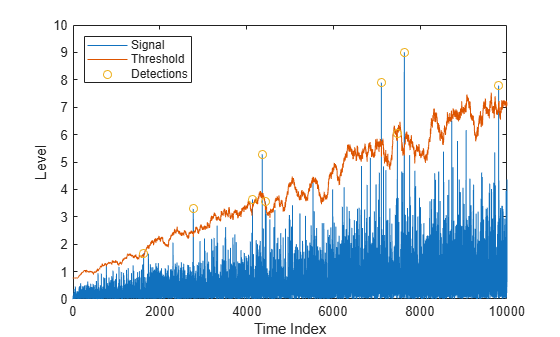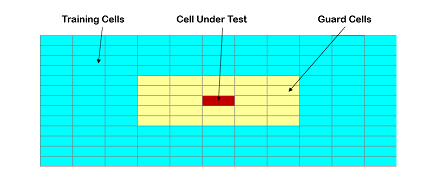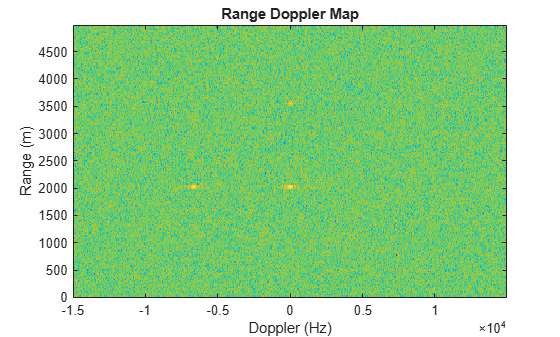Main Content

# Constant False Alarm Rate (CFAR) Detection

This example introduces constant false alarm rate (CFAR) detection and shows how to use CFARDetector and CFARDetector2D in the Phased Array System Toolbox™ to perform cell averaging CFAR detection.

### Introduction

One important task a radar system performs is target detection. The detection itself is fairly straightforward. It compares the signal to a threshold. Therefore, the real work on detection is coming up with an appropriate threshold. In general, the threshold is a function of both the probability of detection and the probability of false alarm.

In many phased array systems, because of the cost associated with a false detection, it is desirable to have a detection threshold that not only maximizes the probability of detection but also keeps the probability of false alarm below a preset level.

There is extensive literature on how to determine the detection threshold. Readers might be interested in the Signal Detection in White Gaussian Noise and Signal Detection Using Multiple Samples examples for some well known results. However, all these classical results are based on theoretical probabilities and are limited to white Gaussian noise with known variance (power). In real applications, the noise is often colored and its power is unknown.

CFAR technology addresses these issues. In CFAR, when the detection is needed for a given cell, often termed as the cell under test (CUT), the noise power is estimated from neighboring cells. Then the detection threshold, $T$, is given by

`$T=\alpha {P}_{n}$`

where ${P}_{n}$ is the noise power estimate and $\alpha$ is a scaling factor called the threshold factor.

From the equation, it is clear that the threshold adapts to the data. It can be shown that with the appropriate threshold factor, $\alpha$, the resulting probability of false alarm can be kept at a constant, hence the name CFAR.

### Cell Averaging CFAR Detection

The cell averaging CFAR detector is probably the most widely used CFAR detector. It is also used as a baseline comparison for other CFAR techniques. In a cell averaging CFAR detector, noise samples are extracted from both leading and lagging cells (called training cells) around the CUT. The noise estimate can be computed as 

`${P}_{n}=\frac{1}{N}\sum _{m=1}^{N}{x}_{m}$`

where $N$ is the number of training cells and ${x}_{m}$ is the sample in each training cell. If ${x}_{m}$ happens to be the output of a square law detector, then ${P}_{n}$ represents the estimated noise power. In general, the number of leading and lagging training cells are the same. Guard cells are placed adjacent to the CUT, both and leading and lagging it. The purpose of these guard cells is to avoid signal components from leaking into the training cell, which could adversely affect the noise estimate.

The following figure shows the relation among these cells for the 1-D case.With the above cell averaging CFAR detector, assuming the data passed into the detector is from a single pulse, i.e., no pulse integration involved, the threshold factor can be written as 

`$\alpha =N\left({P}_{fa}^{-1/N}-1\right)$`

where ${P}_{fa}$ is the desired false alarm rate.

### CFAR Detection Using Automatic Threshold Factor

In the rest of this example, we show how to use Phased Array System Toolbox to perform a cell averaging CFAR detection. For simplicity and without losing any generality, we still assume that the noise is white Gaussian. This enables the comparison between the CFAR and classical detection theory.

We can instantiate a CFAR detector using the following command

`cfar = phased.CFARDetector('NumTrainingCells',20,'NumGuardCells',2);`

In this detector we use 20 training cells and 2 guard cells in total. This means that there are 10 training cells and 1 guard cell on each side of the CUT. As mentioned above, if we assume that the signal is from a square law detector with no pulse integration, the threshold can be calculated based on the number of training cells and the desired probability of false alarm. Assuming the desired false alarm rate is 0.001, we can configure the CFAR detector as follows so that this calculation can be carried out.

```exp_pfa = 1e-3; cfar.ThresholdFactor = 'Auto'; cfar.ProbabilityFalseAlarm = exp_pfa;```

The configured CFAR detector is shown below.

`cfar`
```cfar = phased.CFARDetector with properties: Method: 'CA' NumGuardCells: 2 NumTrainingCells: 20 ThresholdFactor: 'Auto' ProbabilityFalseAlarm: 1.0000e-03 OutputFormat: 'CUT result' ThresholdOutputPort: false NoisePowerOutputPort: false ```

We now simulate the input data. Since the focus is to show that the CFAR detector can keep the false alarm rate under a certain value, we just simulate the noise samples in those cells. Here are the settings:

• The data sequence is 23 samples long, and the CUT is cell 12. This leaves 10 training cells and 1 guard cell on each side of the CUT.

• The false alarm rate is calculated using 100 thousand Monte Carlo trials

```rs = RandStream('mt19937ar','Seed',2010); npower = db2pow(-10); % Assume 10dB SNR ratio Ntrials = 1e5; Ncells = 23; CUTIdx = 12; % Noise samples after a square law detector rsamp = randn(rs,Ncells,Ntrials)+1i*randn(rs,Ncells,Ntrials); x = abs(sqrt(npower/2)*rsamp).^2;```

To perform the detection, pass the data through the detector. In this example, there is only one CUT, so the output is a logical vector containing the detection result for all the trials. If the result is true, it means that a target is present in the corresponding trial. In our example, all detections are false alarms because we are only passing in noise. The resulting false alarm rate can then be calculated based on the number of false alarms and the number of trials.

```x_detected = cfar(x,CUTIdx); act_pfa = sum(x_detected)/Ntrials```
```act_pfa = 9.4000e-04 ```

The result shows that the resulting probability of false alarm is below 0.001, just as we specified.

### CFAR Detection Using Custom Threshold Factor

As explained in the earlier part of this example, there are only a few cases in which the CFAR detector can automatically compute the appropriate threshold factor. For example, using the previous scenario, if we employ a 10-pulses noncoherent integration before the data goes into the detector, the automatic threshold can no longer provide the desired false alarm rate.

```npower = db2pow(-10); % Assume 10dB SNR ratio xn = 0; for m = 1:10 rsamp = randn(rs,Ncells,Ntrials)+1i*randn(rs,Ncells,Ntrials); xn = xn + abs(sqrt(npower/2)*rsamp).^2; % noncoherent integration end x_detected = cfar(xn,CUTIdx); act_pfa = sum(x_detected)/Ntrials```
```act_pfa = 0 ```

One may be puzzled why we think a resulting false alarm rate of 0 is worse than a false alarm rate of 0.001. After all, isn't a false alarm rate of 0 a great thing? The answer to this question lies in the fact that when the probability of false alarm is decreased, so is the probability of detection. In this case, because the true false alarm rate is far below the allowed value, the detection threshold is set too high. The same probability of detection can be achieved with our desired probability of false alarm at lower cost; for example, with lower transmitter power.

In most cases, the threshold factor needs to be estimated based on the specific environment and system configuration. We can configure the CFAR detector to use a custom threshold factor, as shown below.

```release(cfar); cfar.ThresholdFactor = 'Custom';```

Continuing with the pulse integration example and using empirical data, we found that we can use a custom threshold factor of 2.35 to achieve the desired false alarm rate. Using this threshold, we see that the resulting false alarm rate matches the expected value.

```cfar.CustomThresholdFactor = 2.35; x_detected = cfar(xn,CUTIdx); act_pfa = sum(x_detected)/Ntrials```
```act_pfa = 9.6000e-04 ```

### CFAR Detection Threshold

A CFAR detection occurs when the input signal level in a cell exceeds the threshold level. The threshold level for each cell depends on the threshold factor and the noise power in that derived from training cells. To maintain a constant false alarm rate, the detection threshold will increase or decrease in proportion to the noise power in the training cells. Configure the CFAR detector to output the threshold used for each detection using the `ThresholdOutputPort` property. Use an automatic threshold factor and 200 training cells.

```release(cfar); cfar.ThresholdOutputPort = true; cfar.ThresholdFactor = 'Auto'; cfar.NumTrainingCells = 200;```

Next, create a square-law input signal with increasing noise power.

```rs = RandStream('mt19937ar','Seed',2010); Npoints = 1e4; rsamp = randn(rs,Npoints,1)+1i*randn(rs,Npoints,1); ramp = linspace(1,10,Npoints)'; xRamp = abs(sqrt(npower*ramp./2).*rsamp).^2;```

Compute detections and thresholds for all cells in the signal.

`[x_detected,th] = cfar(xRamp,1:length(xRamp));`

Next, compare the CFAR threshold to the input signal.

```plot(1:length(xRamp),xRamp,1:length(xRamp),th,... find(x_detected),xRamp(x_detected),'o') legend('Signal','Threshold','Detections','Location','Northwest') xlabel('Time Index') ylabel('Level')```Here, the threshold increases with the noise power of the signal to maintain the constant false alarm rate. Detections occur where the signal level exceeds the threshold.

### Comparison Between CFAR and Classical Neyman-Pearson Detector

In this section, we compare the performance of a CFAR detector with the classical detection theory using the Neyman-Pearson principle. Returning to the first example and assuming the true noise power is known, the theoretical threshold can be calculated as

`T_ideal = npower*db2pow(npwgnthresh(exp_pfa));`

The false alarm rate of this classical Neyman-Pearson detector can be calculated using this theoretical threshold.

`act_Pfa_np = sum(x(CUTIdx,:)>T_ideal)/Ntrials`
```act_Pfa_np = 9.5000e-04 ```

Because we know the noise power, classical detection theory also produces the desired false alarm rate. The false alarm rate achieved by the CFAR detector is similar.

```release(cfar); cfar.ThresholdOutputPort = false; cfar.NumTrainingCells = 20; x_detected = cfar(x,CUTIdx); act_pfa = sum(x_detected)/Ntrials```
```act_pfa = 9.4000e-04 ```

Next, assume that both detectors are deployed to the field and that the noise power is 1 dB more than expected. In this case, if we use the theoretical threshold, the resulting probability of false alarm is four times more than what we desire.

```npower = db2pow(-9); % Assume 9dB SNR ratio rsamp = randn(rs,Ncells,Ntrials)+1i*randn(rs,Ncells,Ntrials); x = abs(sqrt(npower/2)*rsamp).^2; act_Pfa_np = sum(x(CUTIdx,:)>T_ideal)/Ntrials```
```act_Pfa_np = 0.0041 ```

On the contrary, the CFAR detector's performance is not affected.

```x_detected = cfar(x,CUTIdx); act_pfa = sum(x_detected)/Ntrials```
```act_pfa = 0.0011 ```

Hence, the CFAR detector is robust to noise power uncertainty and better suited to field applications.

Finally, use a CFAR detection in the presence of colored noise. We first apply the classical detection threshold to the data.

```npower = db2pow(-10); fcoeff = maxflat(10,'sym',0.2); x = abs(sqrt(npower/2)*filter(fcoeff,1,rsamp)).^2; % colored noise act_Pfa_np = sum(x(CUTIdx,:)>T_ideal)/Ntrials```
```act_Pfa_np = 0 ```

Note that the resulting false alarm rate cannot meet the requirement. However, using the CFAR detector with a custom threshold factor, we can obtain the desired false alarm rate.

```release(cfar); cfar.ThresholdFactor = 'Custom'; cfar.CustomThresholdFactor = 12.85; x_detected = cfar(x,CUTIdx); act_pfa = sum(x_detected)/Ntrials```
```act_pfa = 0.0010 ```

### CFAR Detection For Range-Doppler Images

In the previous sections, the noise estimate was computed from training cells leading and lagging the CUT in a single dimension. We can also perform CFAR detection on images. Cells correspond to pixels in the images, and guard cells and training cells are placed in bands around the CUT. The detection threshold is computed from cells in the rectangular training band around the CUT.In the figure above, the guard band size is [2 2] and the training band size is [4 3]. The size indices refer to the number of cells on each side of the CUT in the row and columns dimensions, respectively. The guard band size can also be defined as 2, since the size is the same along row and column dimensions.

Next, create a two-dimensional CFAR detector. Use a probability of false alarm of 1e-5 and specify a guard band size of 5 cells and a training band size of 10 cells.

```cfar2D = phased.CFARDetector2D('GuardBandSize',5,'TrainingBandSize',10,... 'ProbabilityFalseAlarm',1e-5);```

Next, load and plot a range-doppler image. The image includes returns from two stationary targets and one target moving away from the radar.

`[resp,rngGrid,dopGrid] = helperRangeDoppler;`Use CFAR to search the range-doppler space for objects, and plot a map of the detections. Search from -10 to 10 kHz and from 1000 to 4000 m. First, define the cells under test for this region.

```[~,rangeIndx] = min(abs(rngGrid-[1000 4000])); [~,dopplerIndx] = min(abs(dopGrid-[-1e4 1e4])); [columnInds,rowInds] = meshgrid(dopplerIndx(1):dopplerIndx(2),... rangeIndx(1):rangeIndx(2)); CUTIdx = [rowInds(:) columnInds(:)]';```

Compute a detection result for each cell under test. Each pixel in the search region is a cell in this example. Plot a map of the detection results for the range-doppler image.

```detections = cfar2D(resp,CUTIdx); helperDetectionsMap(resp,rngGrid,dopGrid,rangeIndx,dopplerIndx,detections)```The three objects are detected. A data cube of range-doppler images over time can likewise be provided as the input signal to `cfar2D`, and detections will be calculated in a single step.

### Summary

In this example, we presented the basic concepts behind CFAR detectors. In particular, we explored how to use the Phased Array System Toolbox to perform cell averaging CFAR detection on signals and range-doppler images. The comparison between the performance offered by a cell averaging CFAR detector and a detector equipped with the theoretically calculated threshold shows clearly that the CFAR detector is more suitable for real field applications.

### Reference

 Mark Richards, Fundamentals of Radar Signal Processing, McGraw Hill, 2005

## Support

#### Exploring Hybrid Beamforming Architectures for 5G Systems

Download white paper